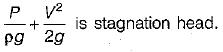Courses

# Flow Through Pipes - 1

## 10 Questions MCQ Test Topicwise Question Bank for GATE Civil Engineering | Flow Through Pipes - 1

Description
This mock test of Flow Through Pipes - 1 for Civil Engineering (CE) helps you for every Civil Engineering (CE) entrance exam. This contains 10 Multiple Choice Questions for Civil Engineering (CE) Flow Through Pipes - 1 (mcq) to study with solutions a complete question bank. The solved questions answers in this Flow Through Pipes - 1 quiz give you a good mix of easy questions and tough questions. Civil Engineering (CE) students definitely take this Flow Through Pipes - 1 exercise for a better result in the exam. You can find other Flow Through Pipes - 1 extra questions, long questions & short questions for Civil Engineering (CE) on EduRev as well by searching above.
QUESTION: 1

Solution:
QUESTION: 2

Solution:
QUESTION: 3

### Consider the following conditions for the pipe network shown in the given figure (Notations have the usual meaning with suffixes 1, 2 and 3 referring to respective pipes):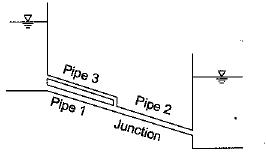1. Q1 = Q3 2. Q2 = Q1 + Q3 3. hf1 = hf3 4. hf1 = hf2 = hf3 Which of these conditions must be satisfied by this pipe network?

Solution:
QUESTION: 4

Maximum pressure rise due to water hammer in a pipeline (a = area of the pipe; V0 = Velocity, g = acceleration due to gravity; t = time period; L = length of the pipeline) is

Solution:

For instantaneous closure of valve, the pressure head at valve end is,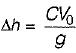Time period for travel of pressure wave from one end to the other is,
t = L/C
Therefore velocity of pressure wave,
C = L/t
and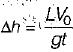QUESTION: 5

Water of v = 1 centiStoke flows through a 1 cm diameter pipe. Critical flow will correspond to a discharge of approximately

Solution:

Rec = 2000 For critical flow
Viscosity, v = 0.01 Stokes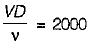Velocity of flow,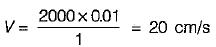Flow at critical Reynolds number,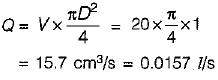QUESTION: 6

A penstock is 2000 m long and the velocity of pressure wave in it is 1000 m/s. Water hammer pressure head for instantaneous closure of valve at the downstream end of the pipe is 60 m. If the valve is closed in 4 s, then what is the peak water hammer pressure in m of water?

Solution:

We know that critical time is given by,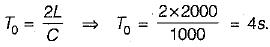Actual time for valve closure, T = 4s.
We know that if T < T0, then the closure is known as rapid closure or instantaneous closure. Therefore the peak water hammer pressure will be equal to the water hammer pressure head for instantaneous closure of the valve at the downstream end i.e. 60 m.

QUESTION: 7

The power transmitted through a pipeline is maximum when the head lost due to friction in the pipe is equal to

Solution:

If H is the total supply head and hf is the loss of head due to friction, then head available at the outlet of pipe (neglecting the minor losses) is H - hf. If Q is the discharge through the pipe , V is the velocity of flow and L and D are the length and the diameter of the pipe respectively, then from Darcy-Weisbach equation we have,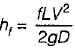and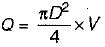The power available at the outlet of pipeline is given by,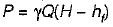⇒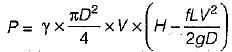For a given pipe and head at the entrance, the condition for the maximum power transmitted through the pipe line may be obtained by differentiating Pw.r.t V and equating it to zero.
∴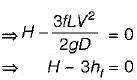⇒ hf = H/3

QUESTION: 8

The relationship dp/dx = dζ/dy is valid for

Solution: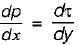is valid for a steady uniform laminar flow.

QUESTION: 9

Water hammer wave velocity through a rigid pipe conveying sea water with specific weight = 10065 N/m3 and bulk modulus of elasticity = 1.96 x 109 N/m2 is

Solution: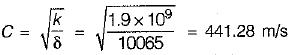QUESTION: 10

The stagnation pressure is the sum of

Solution: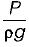is pressure head or static head.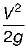is velocity head or dynamic head or kinetic head.